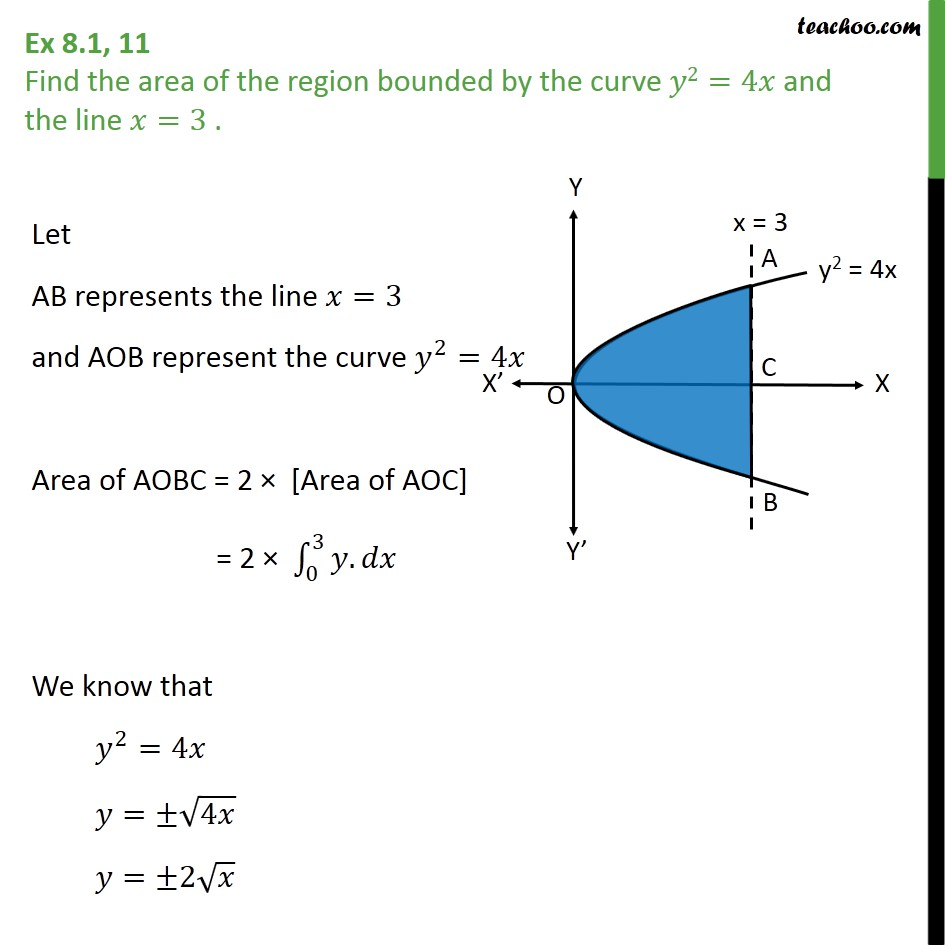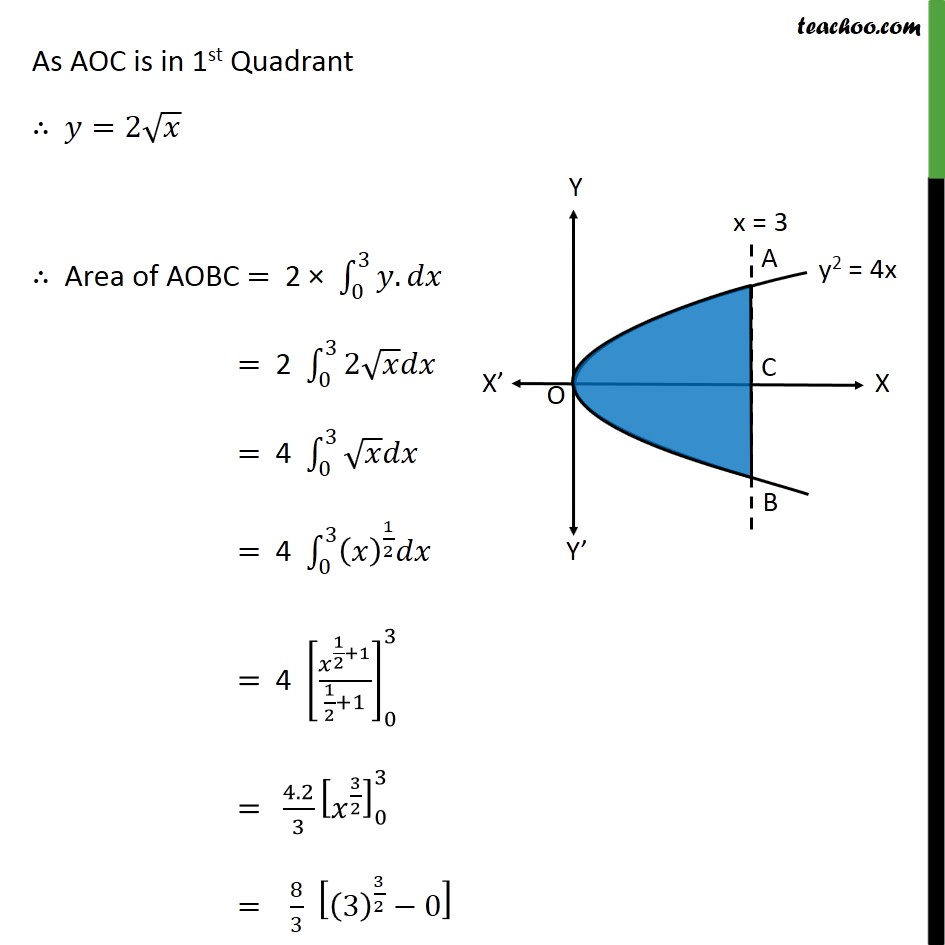Area bounded by curve and horizontal or vertical line

Chapter 8 Class 12 Application of Integrals
Concept wiseLearn in your speed, with individual attention - Teachoo Maths 1-on-1 Class

### Transcript

Question 9 Find the area of the region bounded by the curve 2=4 and the line =3 . Let AB represents the line =3 and AOB represent the curve 2 =4 Area of AOBC = 2 [Area of AOC] = 2 0 3 . We know that 2 =4 = 4 = 2 As AOC is in 1st Quadrant =2 Area of AOBC = 2 0 3 . = 2 0 3 2 = 4 0 3 = 4 0 3 1 2 = 4 1 2 +1 1 2 +1 0 3 = 4.2 3 3 2 0 3 = 8 3 3 3 2 0 = 8 3 3 3 2 0 = 8 3 3 3 = 8 3 3 3 3 = 8 3 3 3 = 8 3 Required Area = square units